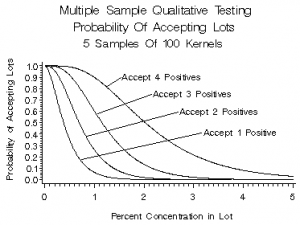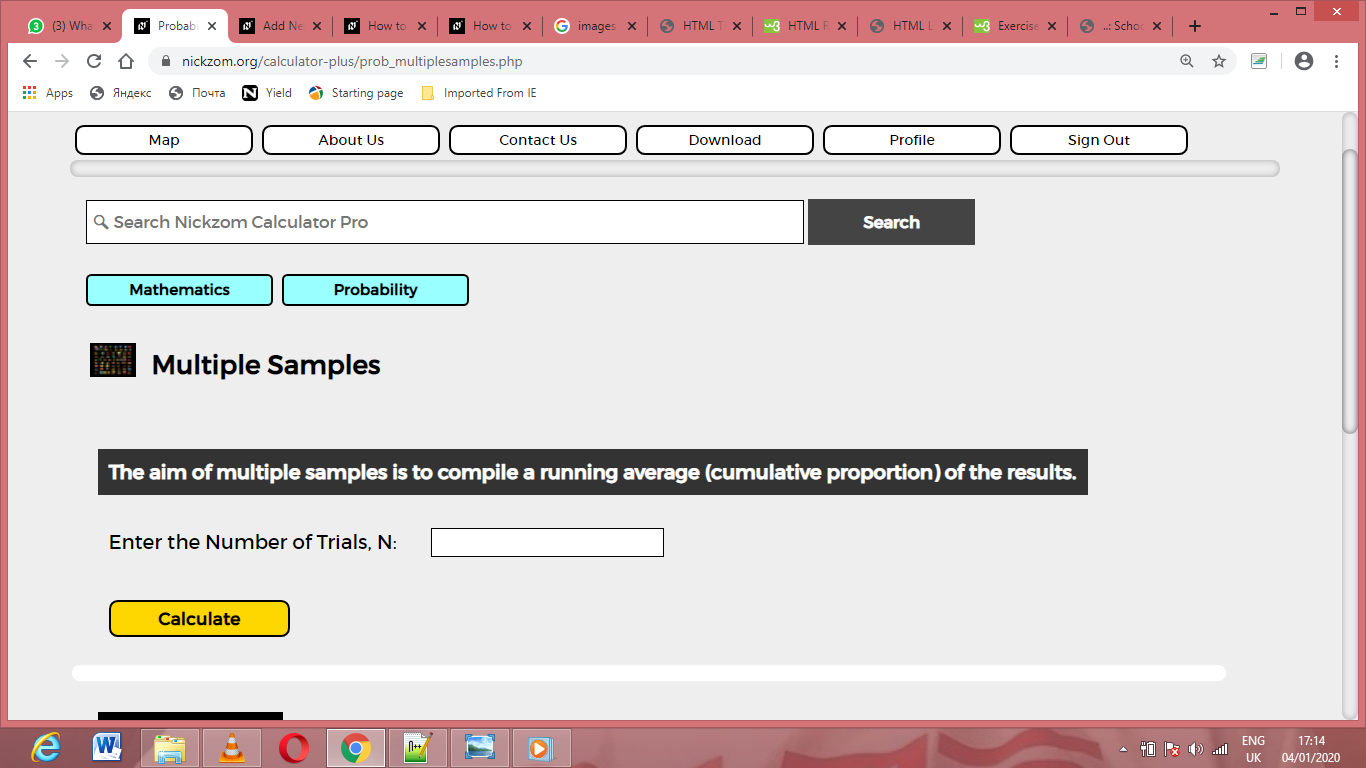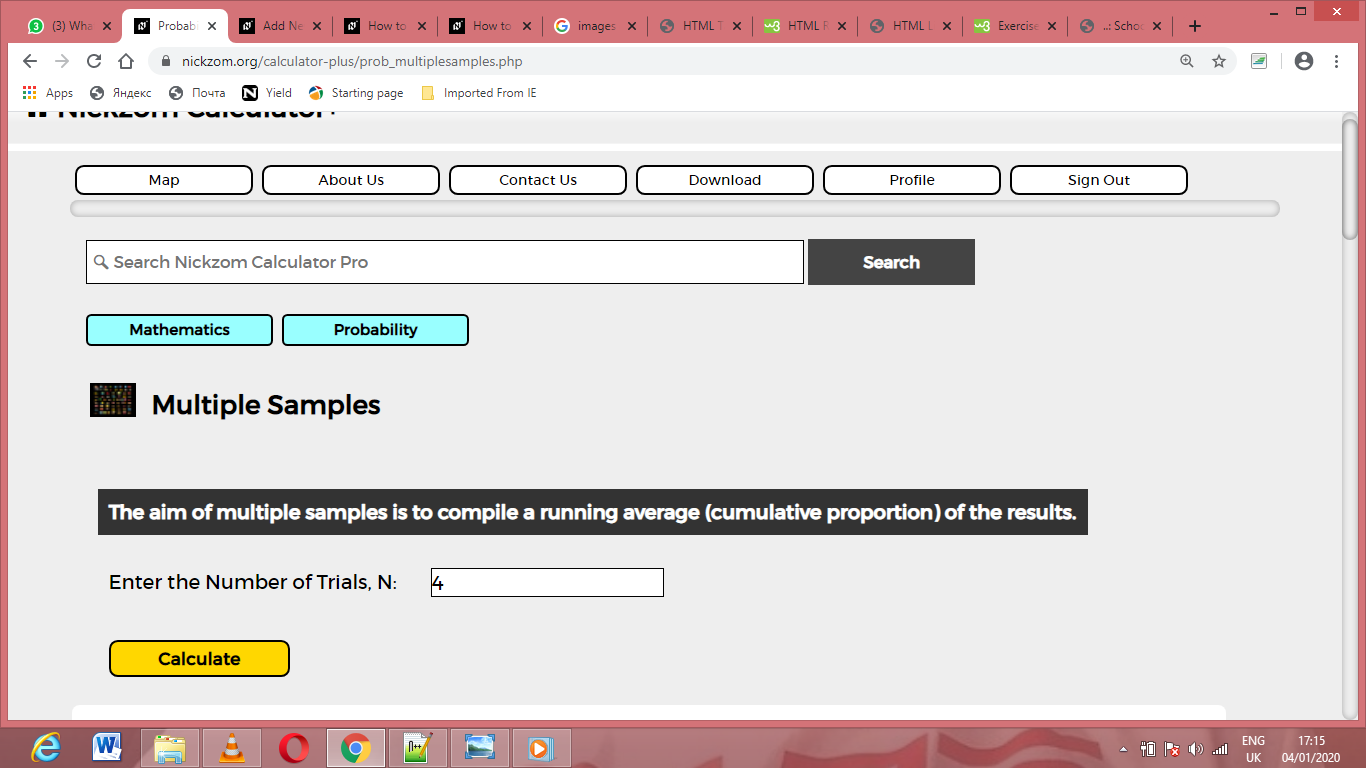# How to Calculate and Solve for Multiple Samples | ProbabilityThe image above represents multiple samples.

To compute for multiple samples, one essential parameter is needed and this parameter is Number of Trials (N).

If Number of Trials (N) is 4.

N      x

1      10
2       5
3       2
4       1

The multiple samples will be calculated:

N       x      Cum x        Running average

1       10        10                 10.0
2        5         15                 7.50
3        2         17                 5.67
4        1         18                 4.50

Nickzom Calculator – The Calculator Encyclopedia is capable of calculating the multiple samples.

To get the answer and workings of the multiple samples using the Nickzom Calculator – The Calculator Encyclopedia. First, you need to obtain the app.

You can get this app via any of these means:

To get access to the professional version via web, you need to register and subscribe for NGN 1,500 per annum to have utter access to all functionalities.
You can also try the demo version via https://www.nickzom.org/calculator

Apple (Paid) – https://itunes.apple.com/us/app/nickzom-calculator/id1331162702?mt=8
Once, you have obtained the calculator encyclopedia app, proceed to the Calculator Map, then click on Probability under Mathematics.Now, Click on Multiple Samples under ProbabilityThe screenshot below displays the page or activity to enter your value, to get the answer for the multiple samples according to the respective parameter which is the Number of Trial (N).Now, enter the value appropriately and accordingly for the parameter as required by the Number of Trial (N) is 4.Now, enter the number of successes x in each trialFinally, Click on CalculateAs you can see from the screenshot above, Nickzom Calculator– The Calculator Encyclopedia solves for the multiple samples, presents the formula, workings and steps too.